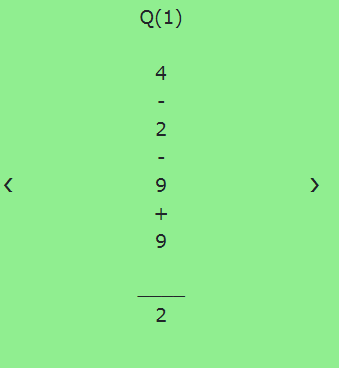# How to display elements of array one by one on clicking next button?

I have tried many solutions but they are not working for my purpose here is my php code which is working fine for generating a random expression.

``````<?php
if(isset(\$_POST['play']))
{
//validation for no of operation selected
\$operation = \$_POST['operation'];
if(empty(\$operation))
{
echo "Please Select at least one operation <br/>";
}
else
{
\$N = count(\$operation); //count selected operation
?>
<div class="slideshow-container" id="slide">
<center>
<?php
echo  "<br />";
echo "This is your generated sequence !<br><br>";
echo "You have selected \$N operation(s):";

for(\$i=0; \$i < \$N; \$i++)  //for loop for for operator array.
{
echo(\$operation[\$i] . ", "); //display selected operation
}
echo "<br />";
?>
</center>
</div>
<?php
//checkbox operations
if(\$N==1)   //if only one operation is selected
{
{
\$rOperators = array('+');
}
else if(\$operation=='Subtraction')
{
\$rOperators = array('-');
}
else if(\$operation=='Multiplication')
{
\$rOperators = array('*');
}
else
{
\$rOperators = array('/');
}
}
else if (\$N==2) //if only two operations are selected by the user
{
{
if(\$operation=='Subtraction')
{
\$rOperators = array('+','-');
}
else if(\$operation=='Multiplication')
{
\$rOperators = array('+','*');
}
else
{
\$rOperators = array('+','/');
}
}
else if(\$operation=='Subtraction')
{
if(\$operation=='Multiplication')
{
\$rOperators = array('-','*');
}
else
{
\$rOperators = array('-','/');
}
}
else
{
\$rOperators = array('*','/');
}
}
else if (\$N==3) //if 3 o
{
{
if(\$operation=='Subtraction')
{
if(\$operation=='Multiplication')
{
\$rOperators = array('+','-','*');
}
else
{
\$rOperators = array('+','-','/');
}

}
else
{
\$rOperators = array('+','*','/');
}
}
else
{
\$rOperators = array('-','*','/');
}
}
else
{
\$rOperators = array('+','-','*','/');
}
}
//display sequence having only single digit numbers
if(\$_POST['digits'] == 1)
{
\$q = \$_POST['que'];
\$previousInt = null;        //Track the previous operand
\$s = \$_POST['select'];

//display inputs given by user through form
?>
<div class="slideshow-container" id="slide">
<center>
<?php
echo "No.of questions:".\$q. "<br />";
echo "No. of integers:" .\$s. "<br />";
echo  "No of digits: 1 <br />";
?>
</center>
<?php
for (\$x = 1; \$x<=\$q; \$x++) //for loop for no. of questions
{
\$randomOperands = array();
\$randomOperators = array();

// loop over \$i n times
for (\$i = 1; \$i <=\$s; \$i++) //for loop for no. of integers user entered
{
\$nextInt = rand(1, 9); // assign random operand to array slot
\$randomOperands[] = \$nextInt;
if(\$i < \$s)
{
if(\$previousInt === 0) //No operator after last opearnd
{
//avoid division-by-zero
\$randomOperators[] = \$rOperators[rand(0, 2)];
}
else
{
\$randomOperators[] = \$rOperators[rand(0, \$N-1)];
}
}
\$previousInt = \$nextInt;
}

// print array values:
\$exp = '';
\$output_string = " ";
//Generating the expression
foreach(\$randomOperands as \$key=>\$value1)
{
\$exp .=  \$value1 . "" ;
\$output_string .= \$value1."<br>";

if(isset(\$randomOperators[\$key]))
{
\$exp .=  \$randomOperators[\$key]  ."";
\$output_string .= \$randomOperators[\$key]."<br>";
}

}
//print expression

\$res = eval("return (\$exp);");//evaluate the expression
\$generate =  array(\$output_string , \$res);
?>
<div class="mySlider">

<!--  back button to go back to previous element of expression-->
<a class="back" id= "pre">❮</a>

<!--display of the expression-->
<?php

echo 'Q('.\$x.')<br><br>'.\$generate.<br>';
?>

<!-- generate result with show button-->
<div class="ansdiv"><?php echo "<br>____<br>'.\$generate."<br>"; ?></div><br>

<!-- next buttonto  go to next element of expression >
<a class="next" id = "fro">❯</a>
</div>
``````

here is screen shot of output I am gettingso as you can see in screenshot the expression is generating with its result, Here `\$output_string` is the expression and `\$res` is result of expression they both are stored in array named `\$generate` Now I want to show the all the elements of array one by one on clicking next button i.e the first element should be 4 according to screenshot and after clicking next button there should be - operator and so on untill the result displayed at last.
NOTE - Here everytime the value of `\$output_string` variable will be different because The expressions are generating randomly and accordingly the result will also changed.this is working fine for my purpose but I tried many solution I am unable somehow to display elements one by one. How can I fix it? Please help me ?

Will the user have the right to cycle through every array-item he wants?

• Take the current array-key from the URL
• validate if it really exists in the array, otherwise take a fallback
• show data to this key
• provide a button to switch to the next array key
• functions: `array_key_exists`, `array_keys`, `next`

Should the user be restricted to move to the next value only?

• start a session first
• calculate the current and next key within your script, store this in the session
• read: `session_start` and the other functions above
1 Like

Hey thankks for the suggestion ,
yes, the user will have the right to cycle through every array-item he wants.
I tried it but its not working . even I have attached previous button to id to go back to previous element if user clicked on back button.

I am not getting clear idea from that suggestion because I am beginner in php .

Thanks for help.
I have updated the code according to the example you have given in previous suggestion.
In my case how will I get the values stored in the variables \$output_string and \$res which are stored in a single array named as \$generate ?
here is my updated code,

``````                                             <?php
\$generate = [\$output_string, \$res];//this is array where all the output i sstored
// Take the current array-key
\$key = 0;

// show data to this key
echo \$elements[\$key];

// provide a button to switch to the next array key
print_r('<a href="?key=%s">></a>', \$key+1);

?>
``````

Where I went wrong?
Here I wnt to display values stored in first variable i.e \$output_string one by one first because it cantains expression and then value stored in \$res which contain result of the expression?

Please tell me where I went wrong?

`\$elements` is undefined, and `\$generate` is not \$output_string

This topic was automatically closed 91 days after the last reply. New replies are no longer allowed.